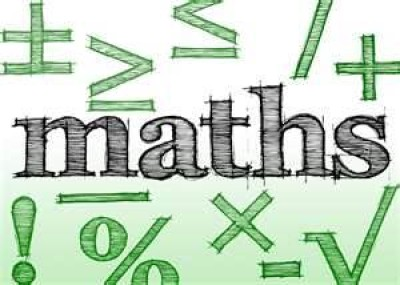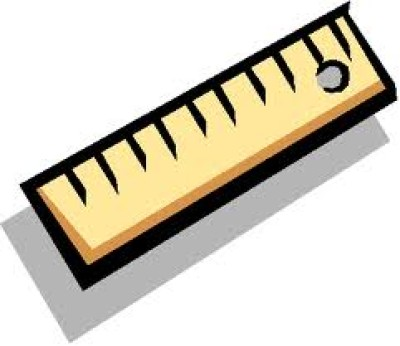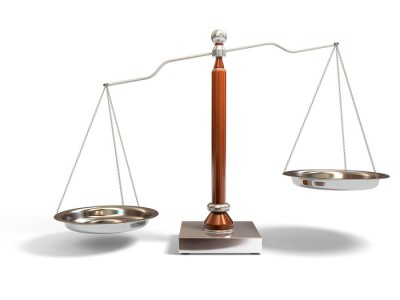List of public pages created with Protopage

Home

Plain sticky notes

Sticky note

Hello welcome to my website. Hope you like it :0)

Calendars

Calendar

• Tue December 25 - CHRISTMAS!!

PhotosBookmarks

Plain sticky notes

Sticky note

Here are some (hopefully) useful bookmarks. You can also find them on my homepage. :0)

Converting measurments

Rich sticky notes

WEIGHT

1000 milligrams = 1 gram

1000 grams       =  1 kilogram
1000 kilograms =  1 tonne

1g           = 0.035 ounces (oz)
1kg         = 2.205 pounds (lb)
1 tonne  = 0.984 tons
1oz        = 28.35 g
1lb         = 0.4536 kg
1 stone   = 6.35 kg
1 ton      = 1.016 tonnes

LENGTH

10    millimetres  = 1 centimetre
100  centimetres =  1 metre
1000 metres       =   1 kilometre

1mm =  0.04 inch (in)
1cm  =   0.4  inch
1m   =   3.28 feet (ft) = 1.09 yards (yds)
1km  =  0.62 miles
1in     =  25.4 mm = 2.54 cm
1ft      =  30.5  cm =  0.305 m
1yd    =  91.4 cm = 0.914 m
1mile = 1.61 km

CAPACITY

10 millilitres   = 1 centilitre (cl)
10 centilitres = 1 decilitre (dl)
10 decilitres  = 1 litre
10 litres         = 1 decalitre (dal)
10 decalitres = 1 hectolitre (hl)

1 ml       =  0.002 pints (pt) = 0.04 fluid ounces (fl oz)
1 fl oz  =  28.4 ml
1 pt      =   568 ml   = 0.568 l
1 l         =  1.76 pts
1 dal     =   2.20 gallons
1 dl       =   0.0176 pt  = 0.35 fl oz
1 gallon =  4.54 l

PhotosIndices

Plain sticky notes

Sticky note

When a number is to the power of 1 it equals that number. A number that is to the power of 0 equals 1. A number to the power of -1 it turns into its reciprical.